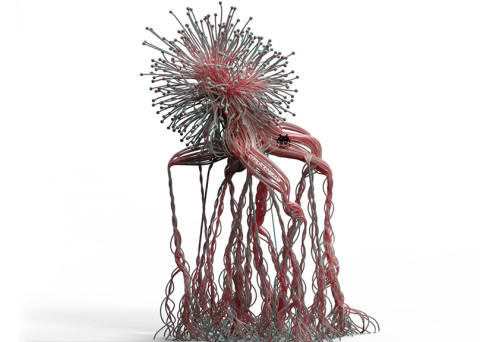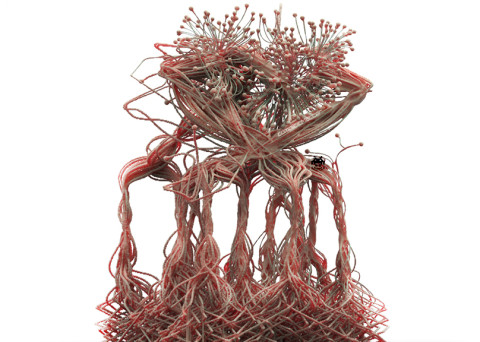# [C]reepyCrawlers

### Gallery details

Code Creates Creepy Crawlers implementing the basic swarm algorithm logic with some fun stuff. – C#

—Quick Study building off of example. Here we have creepy crawlers moving in 2d & 3d. We have implemented the basic swarm algorithm logic to the creepers causing them to turn into creepy crawlers. Each creepy crawler can only see as far as the threshold we provide, which we can visualize by using the connect (link) toggle. We can either contain the creepy crawlers or let them run free as well as run them in 2d or in 3d. Inside the code you can enable or disable spawn types (all on the ground plane, or randomly in the bounding area or along an axis etc). Once again we are using the timer, we are also using data trees to store to previous positions so we can create the trail. This is causing some pretty heavy performance hits once the component has been running for some time. At first it runs very fast however after a few minutes it begins to slow down, I have added a boolean toggle for the trails so you can start the sim and set the trails to false, this will provide a huge performance increase in speed, you can at any time enable it back to true and see the trails and vice versa (if you want the trails to start where the crawlers started then begin the sim with the trails enabled then immediately turn them off as the sim is running, wait however long youd like and turn them back on and it will have kept those previous positions stored and the trail will be created.—

••••••••Using Grasshopper C# components for the code snippets below – All Test code is provided on an ‘as-is’ basis for evaluation, testing and commenting purposes. I am not responsible for any damage incurred by its usage. Use at your own risk.

### CreepyCrawler Class - C# Code

``` //Code written by Luis Quinones @ [complicitMatter]
//Feel free to use and modify the code in any way you want, please comment below with suggestions, ideas of any relevant throughts

private void RunScript(bool reset, int ptCount, int dimension, bool connect, bool swarm, bool bounds, bool trail, double conn_thresh, double moveValue, double searchRad, double aligVal, double sepVal, double cohVal, ref object Creeps, ref object Connectivity, ref object BoundingBox, ref object TrailPoints)
{
Random rnd = new Random();
List<Vector3d> vecs = new List<Vector3d>();
List<Point3d> returnedvecs = new List<Point3d>();

if(reset){ //we are using the reset to reinitialize all the variables and positions to pass to the class once we are running
this.moveList = new List<Vector3d>();
this.startList = new List<Vector3d>();
this.posTree = new DataTree<Point3d>();
this.dimensions = dimension;

if(this.dimensions == 0){
this.bb = new BoundingBox(-250, -250, 0, 250, 250, 0);
}else{
this.bb = new BoundingBox(-250, -250, -250, 250, 250, 500);
}

for(int i = 0; i < ptCount; i++){
if(this.dimensions == 0){ //IF WE WANT 2D
//this.moveVec = new Vector3d(rnd.Next(-1, 2), rnd.Next(-1, 2), 0); //move randomly in any direction 2d
this.moveVec = new Vector3d(moveValue, 0, 0); //move to the right only
//this.startPos = new Vector3d(rnd.Next((int) bb.Min, (int) bb.Max), rnd.Next((int) bb.Min, (int) bb.Max), 0); //spawn randomly inside the bounding area
this.startPos = new Vector3d((int) bb.Min, rnd.Next((int) bb.Min, (int) bb.Max), 0); //spawn along the y axis of the bounding area
}else{ //IF WE WANT 3D
this.moveVec = new Vector3d(rnd.Next(-1, 2), rnd.Next(-1, 2), 0.5); //move randomly in the xy axis and up in the z axis
//this.moveVec = new Vector3d(rnd.Next(-1, 2), rnd.Next(-1, 2), rnd.Next(-1, 2)); //move randomly in the xyz axis
this.moveVec *= moveValue;
//this.startPos = new Vector3d(rnd.Next((int) bb.Min, (int) bb.Max), rnd.Next((int) bb.Min, (int) bb.Max), ((int) bb.Min + (int) bb.Max) / 2); //start on the plane in the middle of the 3d bounds
this.startPos = new Vector3d(rnd.Next((int) bb.Min, (int) bb.Max), rnd.Next((int) bb.Min, (int) bb.Max), (int) bb.Min); //start randomly on the lowest plane of the 3d bounds
//this.startPos = new Vector3d(rnd.Next((int) bb.Min, (int) bb.Max), rnd.Next((int) bb.Min, (int) bb.Max), rnd.Next((int) bb.Min,(int) bb.Max)); //start randomly inside the 3d bounds
}
this.startList.Add(this.startPos); //add the initial starting positions to the list to pass once we start running
this.moveList.Add(this.moveVec); //add the initial move vectors to the list to pass once we start running
}
Creeps = this.startList;
}else{
creepers creeperPts = new creepers(this.startList, this.moveList, bb, this.dimensions, connect, conn_thresh, swarm, searchRad, aligVal, sepVal, cohVal, this.posTree, moveValue, bounds, trail);
creeperPts.action();

this.startList = creeperPts.outputList;
this.moveList = creeperPts.outputMoveList;

Creeps = this.startList;
Connectivity = creeperPts.lineList;
BoundingBox = this.bb;
if(trail){
TrailPoints = creeperPts.posTree;
}
}
}
//-----------------Global Variables---------------------------
private List<Vector3d> moveList = new List<Vector3d>();
private List<Vector3d> startList = new List<Vector3d>();
private DataTree<Point3d>posTree;
private Vector3d startPos = new Vector3d();
private Vector3d moveVec;
private BoundingBox bb;
private int dimensions;

public class creepers{
//-----------------Class Variables------------------------------
private BoundingBox bbox;
public Vector3d pos = new Vector3d();
public Vector3d nextVec = new Vector3d();
public List<Vector3d> posList = new List<Vector3d>();
public List<Vector3d> posMoveList = new List<Vector3d>();
public List<Vector3d> outputList = new List<Vector3d>();
public List<Vector3d> outputMoveList = new List<Vector3d>();
public List<Line> lineList = new List<Line>();
public DataTree<Point3d> posTree;
//-----------Swarm Value fields------------
private bool flag;
private int dim;
private bool conn;
private bool enableBounds;
private bool swarmFlag;
private bool trail;
private double ct;
private double sR;
private double aValue;
private double sValue;
private double cValue;
private double moveAmp;
//-----------Swarm fields------------
private List<Vector3d> otherPtsList;
private List<Vector3d> otherMoveValues;
private List<double> allDist;
private Vector3d cummVec;
//------Swarm behavior fields--------
private Vector3d alignVector;
private Vector3d separateVector;
private Vector3d cohesionVector;
//------------------Constructor----------------------------------
public creepers(List<Vector3d> vecs, List<Vector3d> move, BoundingBox bb, int dimension, bool connect, double connThresh, bool Swarm, double lookArea, double alignVal, double sepVal, double cohVal, DataTree<Point3d> baseTree, double moveVecAmp, bool eb, bool trail){

this.posList = vecs;
this.posMoveList = move;
this.bbox = bb;
this.dim = dimension;
this.conn = connect;
this.ct = connThresh;
this.swarmFlag = Swarm;
this.sR = lookArea;
this.aValue = alignVal;
this.sValue = sepVal;
this.cValue = cohVal;
this.posTree = baseTree;
this.moveAmp = moveVecAmp;
this.enableBounds = eb;
this.trail = trail;
}
//-----------Method Creates the Action----------------------------
public void action(){

for(int i = 0; i < this.posList.Count; i++){ //go through all the creepers
this.pos = new Vector3d();
this.nextVec = new Vector3d();
this.pos = this.posList[i];

if(this.enableBounds){ //check to see if we enabled the bounding area which causes the creeper to turn around if it hits it
this.checkLoc(); //call the checkloc method
if(this.flag){ //if the creeper is on the boundary turn reverse it
Vector3d temp = this.posMoveList[i];
if(this.dim == 0){
if(this.pos.X >= (int) this.bbox.Max || this.pos.X <= (int) this.bbox.Min){
temp.X *= -1;
}
if(this.pos.Y >= (int) this.bbox.Max || this.pos.Y <= (int) this.bbox.Min){
temp.Y *= -1;
}
}else{
if(this.pos.X >= (int) this.bbox.Max || this.pos.X <= (int) this.bbox.Min){
temp.X *= -1;
}
if(this.pos.Y >= (int) this.bbox.Max || this.pos.Y <= (int) this.bbox.Min){
temp.Y *= -1;
}
if(this.pos.Z >= (int) this.bbox.Max || this.pos.Z <= (int) this.bbox.Min){
temp.Z *= -1;
}
}
this.posMoveList[i] = temp;
}
}
this.nextVec = this.posMoveList[i]; //set the move vector the current move vector from the list

if(this.swarmFlag){ //if we want them to interact with each other then enable the feature in the component
swarm();
}
this.pos += this.nextVec; //add the move vec to the current position
GH_Path pth = new GH_Path(i); //set a new path for the data structure from the current count
if(this.trail){
this.posTree.Add((Point3d) this.pos, pth); //add the current position to the data tree at the specified path
}
this.outputMoveList.Add(this.nextVec); //add the next move vec to the output list to return replace the move list
this.outputList.Add(this.pos); //add the new position to the output list to replace the previous current positions

if(this.conn){ //enable link to see how far each creeper can see this will show you their interaction range
}
}
}
//-----------Method Computes the interaction----------------------------
public void swarm(){

this.otherPtsList = new List<Vector3d>();
this.otherMoveValues = new List<Vector3d>();
this.allDist = new List<double>();

for(int i = 0; i < this.posList.Count; i++){
Point3d othercreeper = (Point3d) this.posList[i];
double dist = othercreeper.DistanceTo((Point3d) this.pos);

if(dist > 0 && dist < this.sR){
}
}
if(this.otherPtsList.Count > 0){

this.cummVec = new Vector3d();
//----------Align-----------------
align();
if(this.alignVector.Length > 0){
this.alignVector.Unitize();
}
this.alignVector *= this.aValue;
//----------Separate-----------------
separate();
if(this.separateVector.Length > 0){
this.separateVector.Unitize();
}
this.separateVector *= this.sValue;
//----------Cohesion-----------------
cohesion();
if(this.cohesionVector.Length > 0){
this.cohesionVector.Unitize();
}
this.cohesionVector *= this.cValue;
//-----------------------------------
this.cummVec += this.alignVector;
this.cummVec += this.separateVector;
this.cummVec += this.cohesionVector;
this.nextVec += this.cummVec;
this.nextVec.Unitize();
this.nextVec *= this.moveAmp;
}
}
//-----------Method Computes the alignment----------------------------
public void align(){
this.alignVector = new Vector3d();
for(int i = 0; i < this.otherPtsList.Count; i++){
this.alignVector += (this.otherMoveValues[i] *= (this.sR / this.allDist[i]));
}
}
//-----------Method Computes the separation----------------------------
public void separate(){
this.separateVector = new Vector3d();
for(int i = 0; i < this.otherPtsList.Count; i++){
this.separateVector += Vector3d.Multiply(Vector3d.Subtract(this.pos, this.otherPtsList[i]), this.sR / this.allDist[i]);
}
}
//-----------Method Computes the cohesion-----------------------------
public void cohesion(){
this.cohesionVector = new Vector3d(0, 0, 0);
for(int i = 0; i < this.otherPtsList.Count; i++){
this.cohesionVector += this.otherPtsList[i];
}
Vector3d scaleVec = Vector3d.Multiply(this.cohesionVector, (1.00 / this.otherPtsList.Count));
Point3d posPoint = (Point3d) this.pos;
double dist = posPoint.DistanceTo((Point3d) scaleVec);
this.cohesionVector = Vector3d.Multiply(Vector3d.Subtract(scaleVec, this.pos), this.sR / dist);
}
//-----Method Creates visual diagram of the creeper search space------
for(int i = 0; i < this.outputList.Count; i++){
Point3d othercreeper = (Point3d) this.outputList[i];
double dist = othercreeper.DistanceTo((Point3d) this.pos);

if(dist > 0 && dist < this.ct){
Line l = new Line(othercreeper, (Point3d) this.pos);
}
}
}
//----Method Checks if the creeper is still in the bounding area-----
public void checkLoc(){
flag = new bool();
if(!this.bbox.Contains((Point3d) this.pos)){
//Random rnd = new Random(); //use this if you want the creeper to spawn in a new random area instead of turning it around.
//this.pos = new Vector3d(rnd.Next(-250, 250), rnd.Next(-250, 250), 0); //use this if you want the creeper to spawn in a new random area instead of turning it around.
this.flag = true;
}else{
this.flag = false;
}
}
}
Update
```

• Share